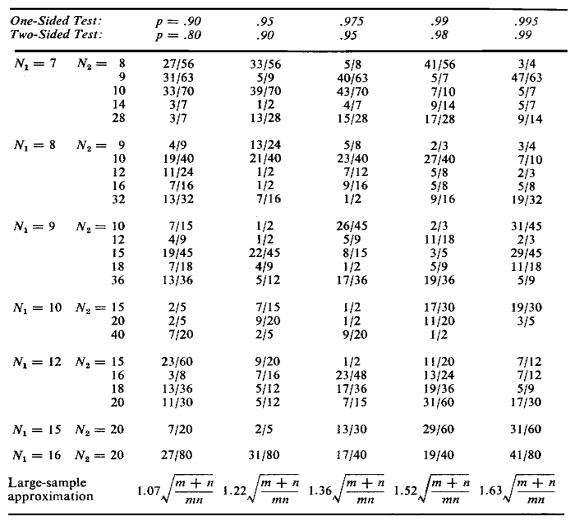Appendix

C

STATISTICAL TABLES

Normal Distribution

Table C-1. Cumulative Probabilities of the Standard Normal Distribution.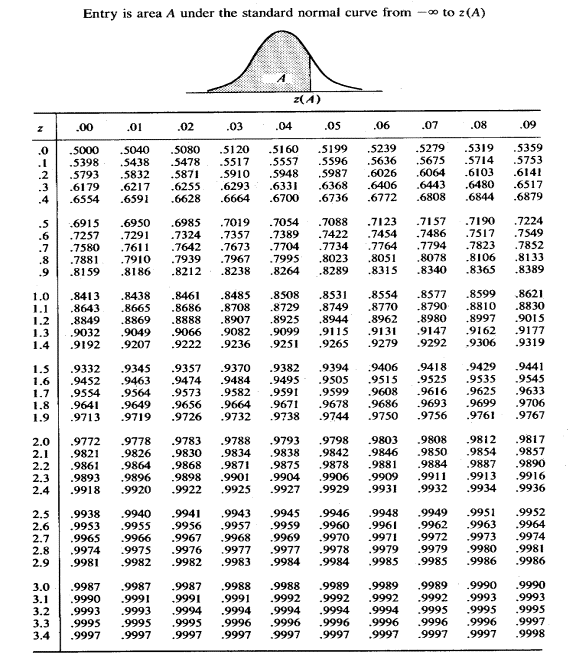Chi-Square Distribution

Table C-2.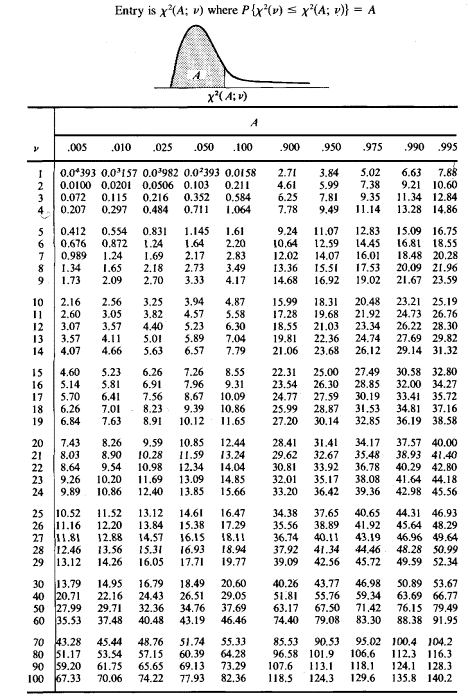Percentiles of the c2 Distribution

Binomial Distribution

Table C-3. Binomial Distribution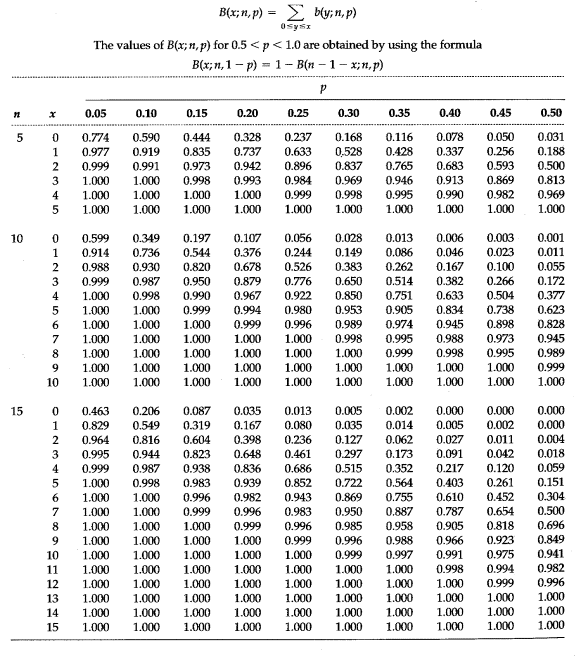Table C-3 (Continued) Binomial Distribution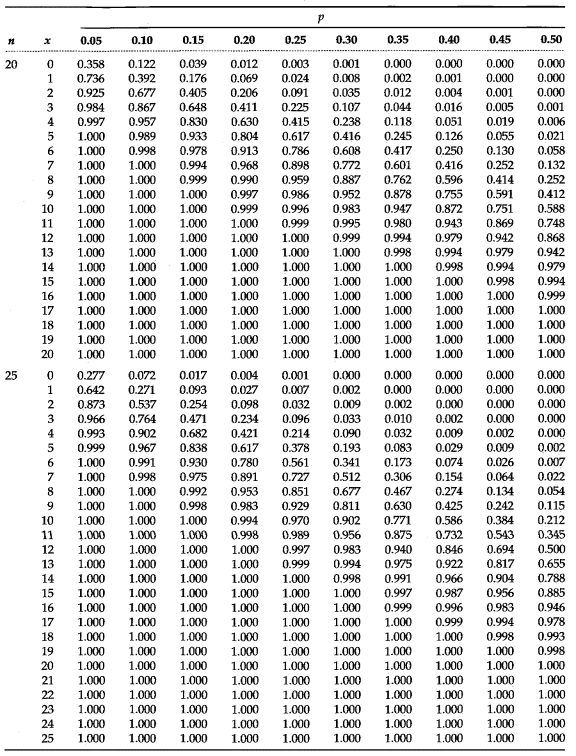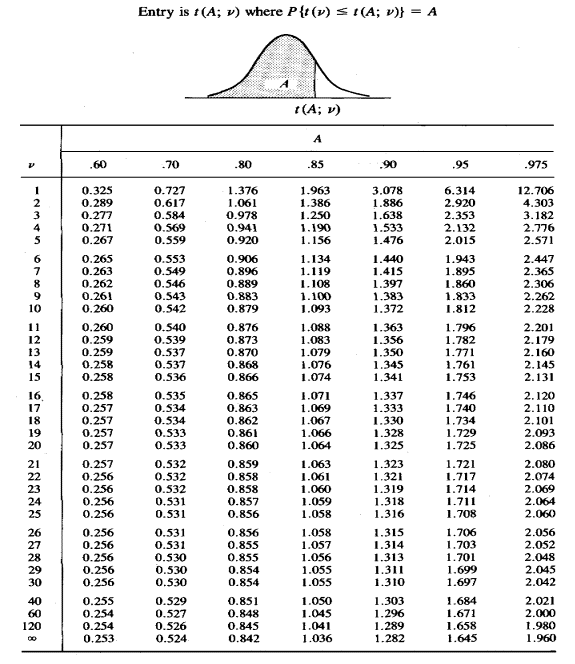Student’s Distribution (t Distribution)

Table C-4 Percentiles of the t Distribution

Table C-4 (Continued) Percentiles of the t Distribution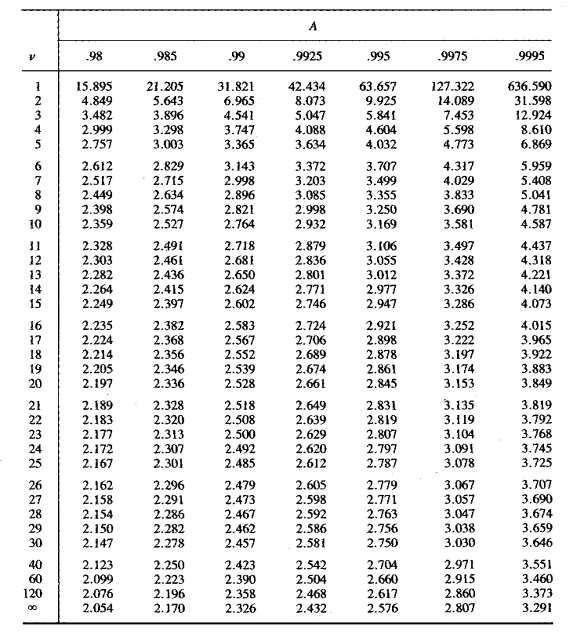F Distribution

Table C-5 Percentiles of the F Distribution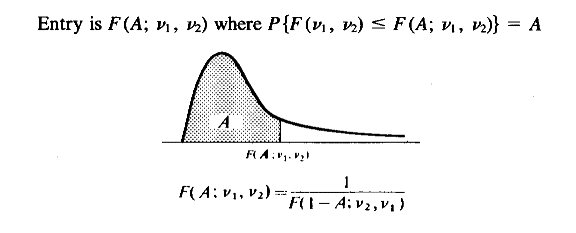Table C-5 (Continued) Percentiles of the F Distribution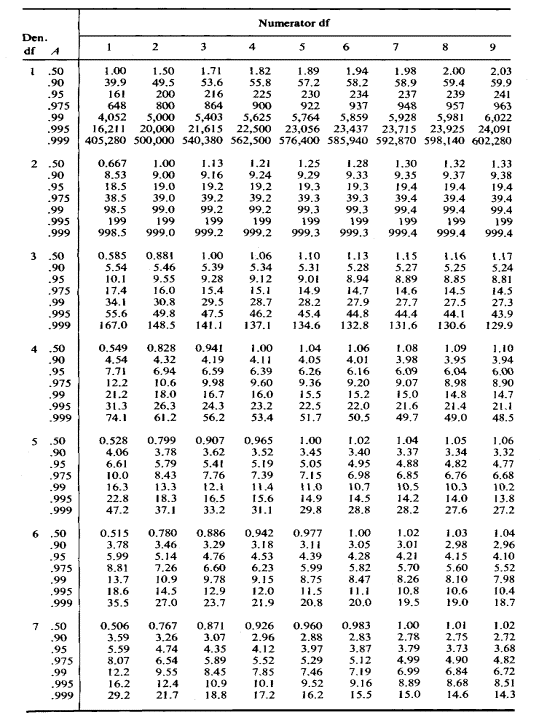Table C-5 (Continued) Percentiles of the F Distribution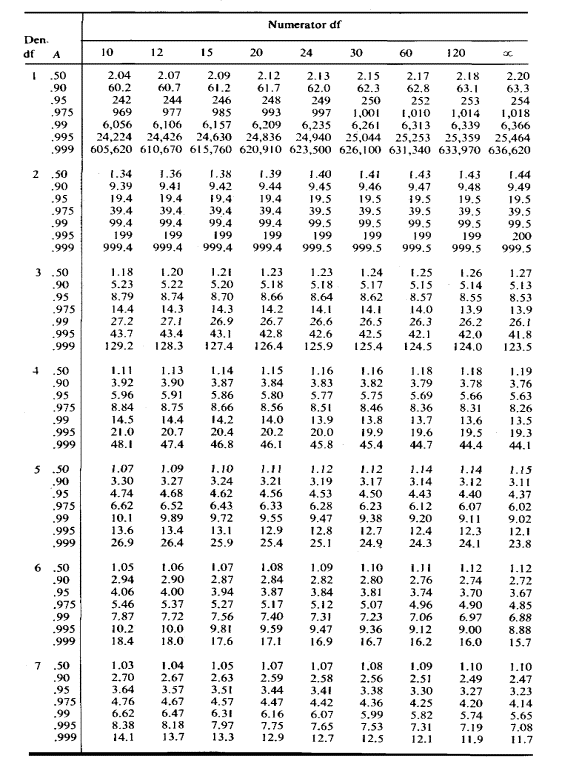Table C-5 (Continued) Percentiles of the F Distribution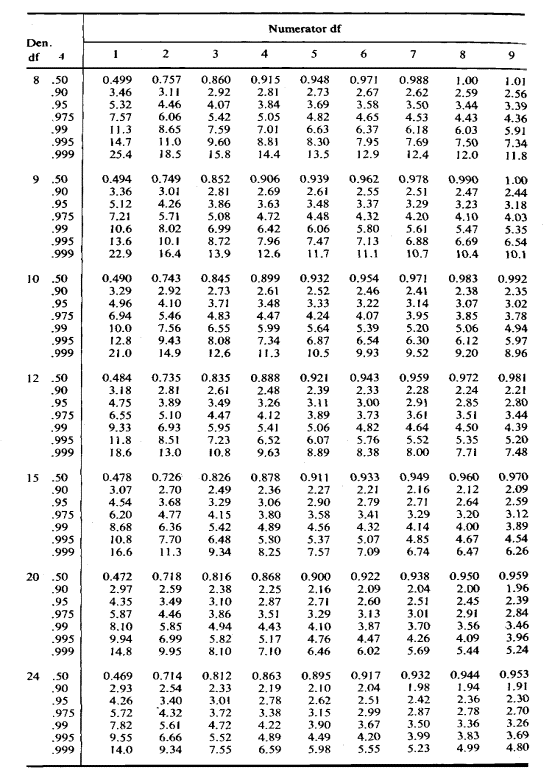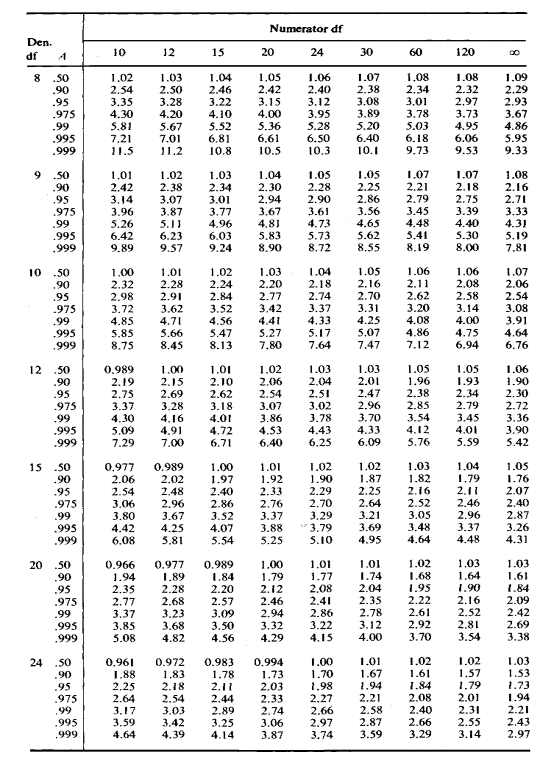Table C-5 (Continued) Percentiles of the F Distribution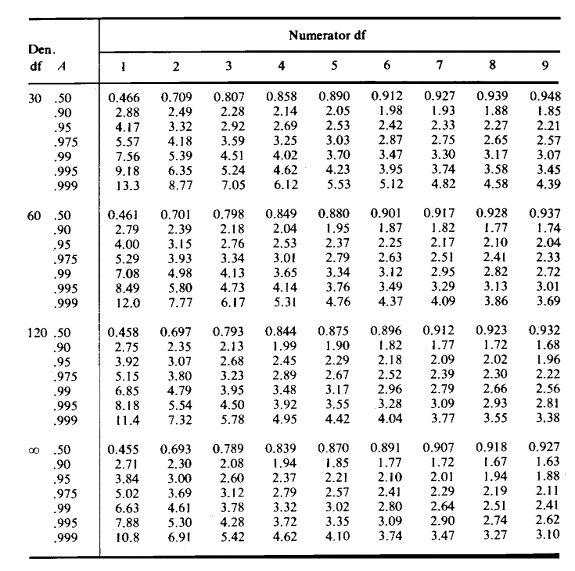Table C-5 (Continued) Percentiles of the F Distribution

Table C-5 (Continued) Percentiles of the F Distribution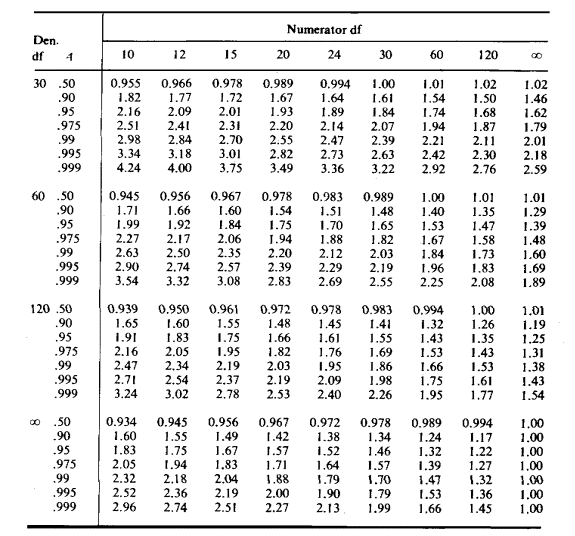Quantiles of the Mann-Whitney Test Statistic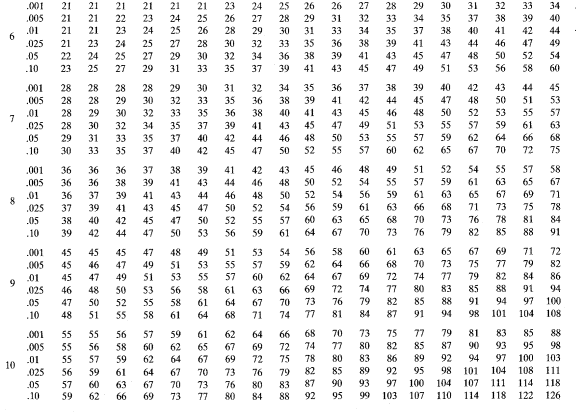Table C-6 Quantiles of the Mann-Whitney Test Statistic

Table C-6 (Continued) Quantiles of the Mann-Whitney Test Statistic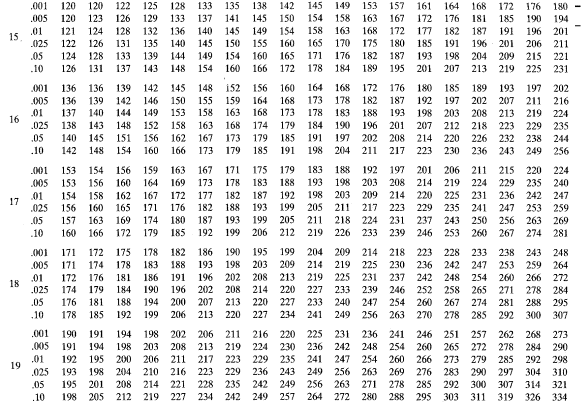Table C-6 (Continued) Quantiles of the Mann-Whitney Test Statistic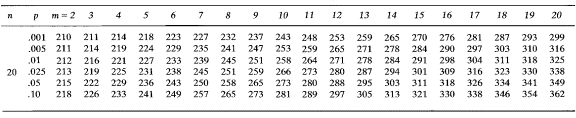Quantiles of the Kruskal-Wallis Test Statistics for Small Sample Sizes

Table C-7 Quantiles of the Kruskal-Wallis Test Statistic for Small Sample Sizes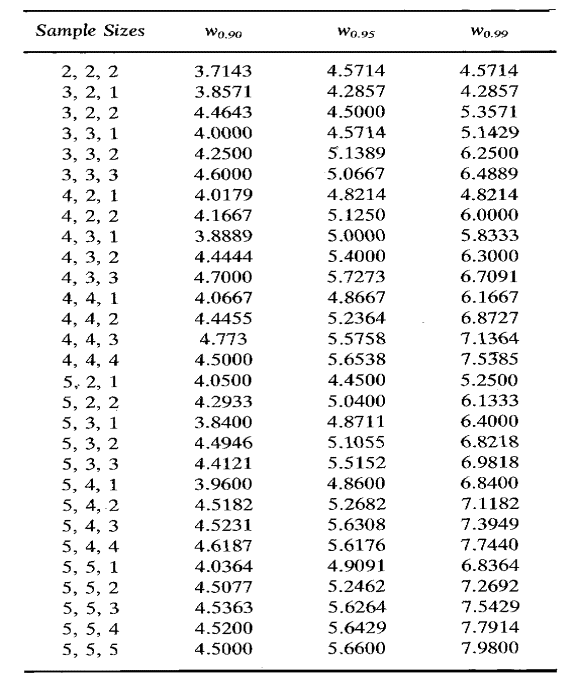Quantiles of the Wilcoxon Signed Rank Test Statistic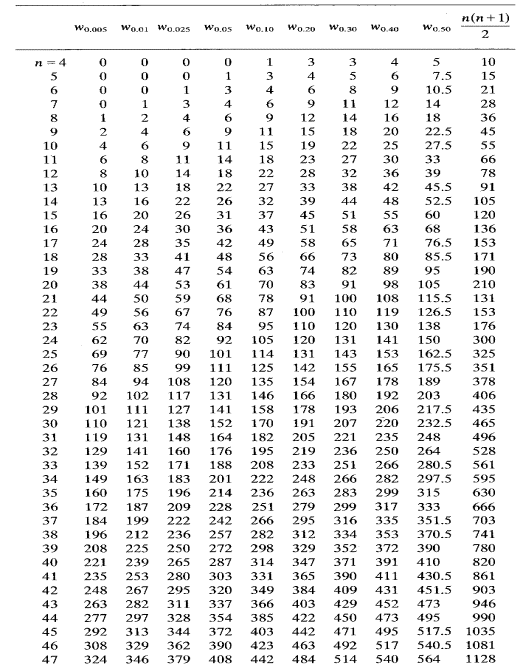Table C-8 Quantiles of the Wilcoxon Signed Ranks Test Statistic

Table C-8 (Continued) Quantiles of the Wilcoxon Signed Ranks Test Statistic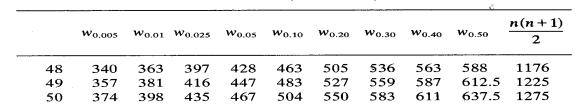For n larger than 50, the pth quantile wp of the Wilcoxon signed ranked test statistic may be approximated by, where xp is the pth quantile of a standard normal random variable, obtained from Table C-1.

Quantiles of Kendall’s Test Statistic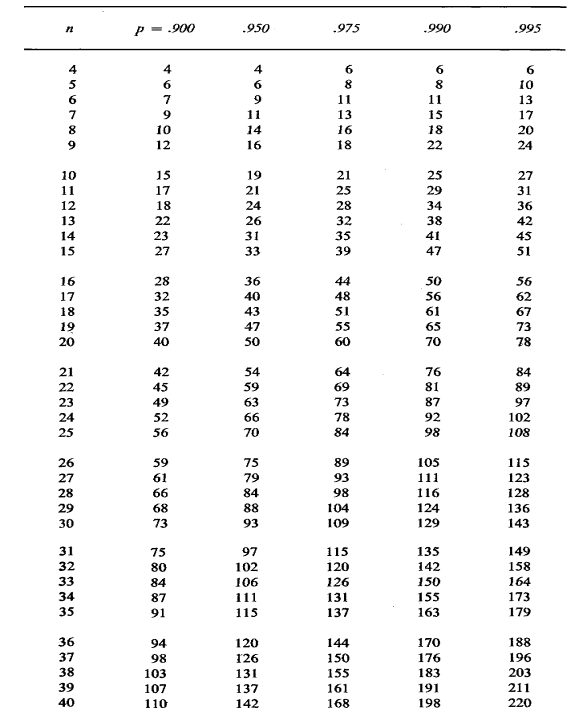Table C-9 Quantiles of the Kendall's Test Statistic

Table C-9 (Continued) Quantiles of the Kendall's Test Statistic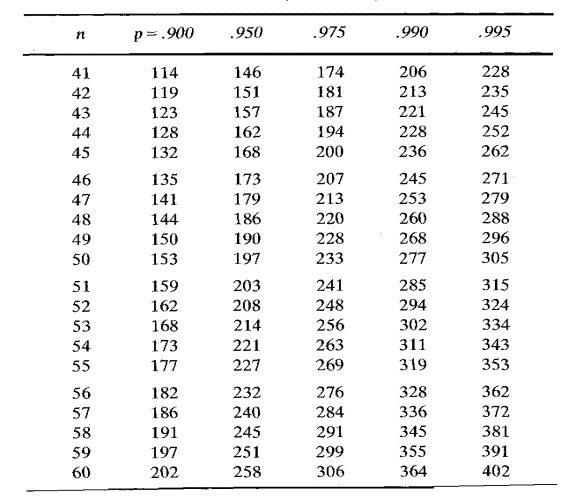Quantiles of the Kilmogorov Test Statistic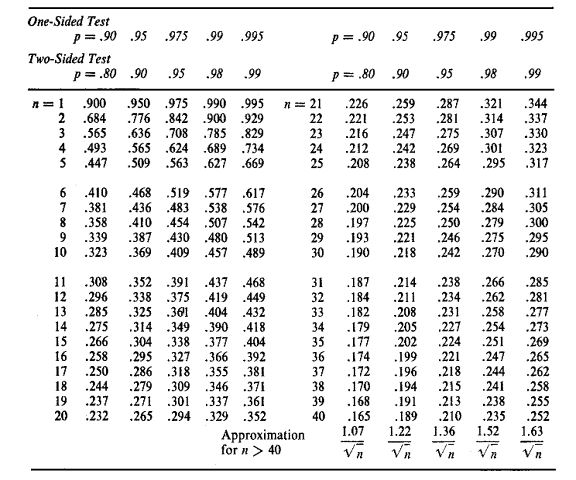Table C-10 Quantiles of the Kilmogorov Test Statistic

Quantiles of the Smirnov Test Statistic for Two Samples of Equal Size

Table C-11 Quantiles of the Smirnov Test Statistic for Two Samples of Equal Size, n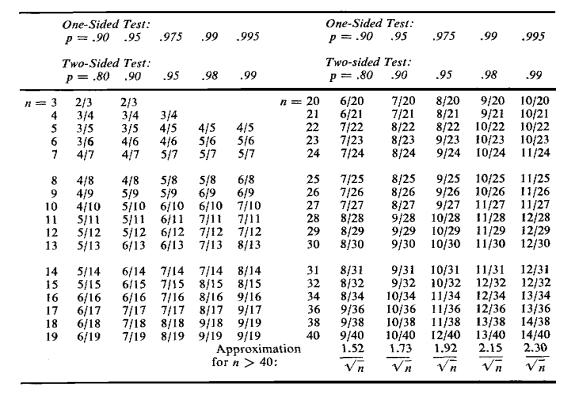Quantiles of the Smirnov Test Statistic for Two Samples of Different Size

Table C-12 Quantiles of the Smirnov Test Statistic for Two Samples of Different Size, n and mTable C-12 (Continued) Quantiles of the Smirnov Test Statistic for Two Samples of Different Size, n and m